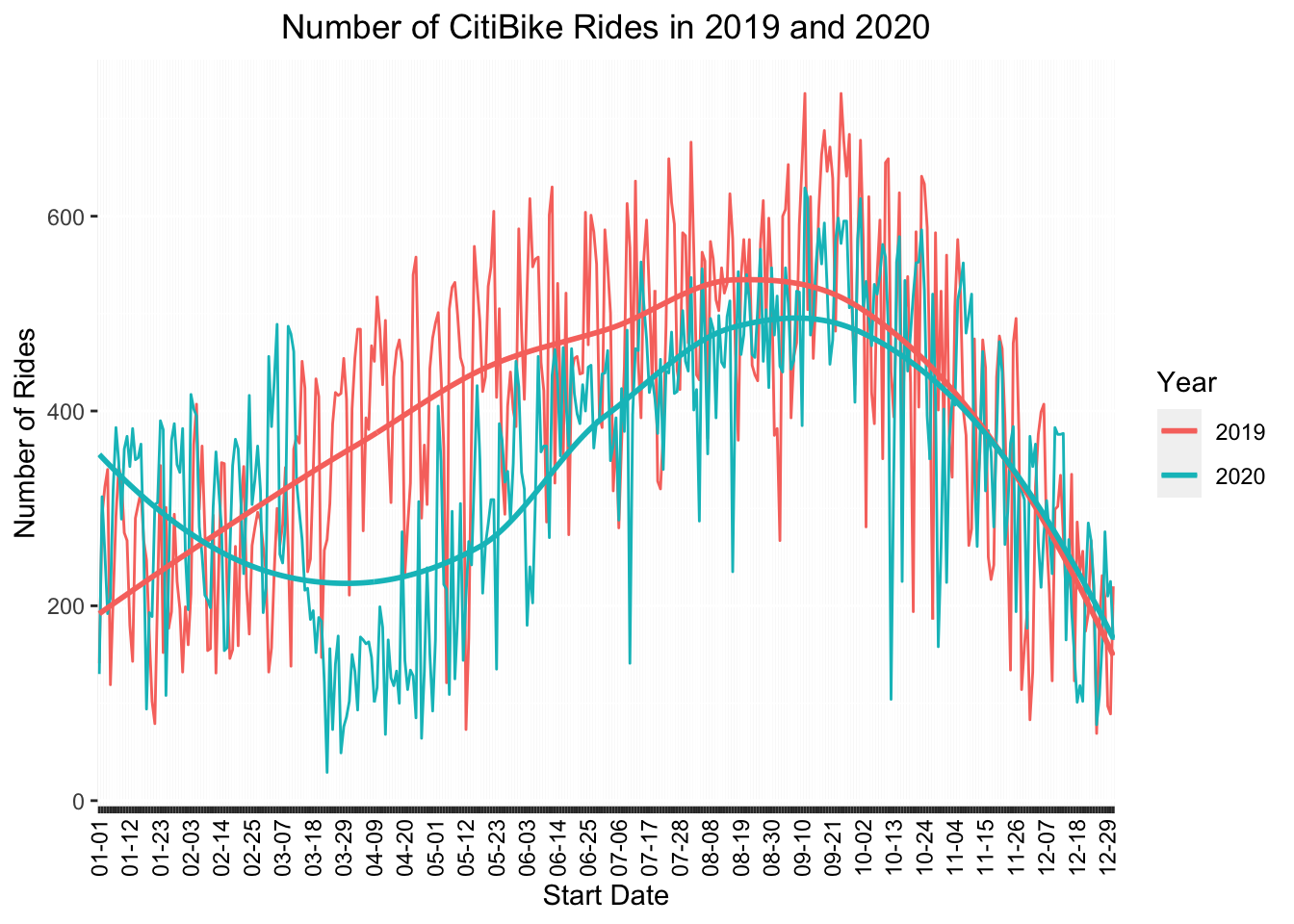``````manhattan_rides_df <- read_csv("manhattan_rides.csv")

manhattan_rides_df <-
manhattan_rides_df %>%
mutate(
day_of_week = factor(day_of_week, ordered = T,
levels = c("Sun", "Mon", "Tue", "Wed", "Thu", "Fri", "Sat")),
year = factor(year),
age_group = factor(age_group, ordered = T,
levels = c("18-25","26-35", "36-45", "46-55", "56-65", "66-85")),
gender = type.convert(gender, as.is = F))``````

## CitiBike Rides Over the Year

``````manhattan_rides_df %>%
group_by(start_date, year) %>%
summarize(obs = n()) %>%
ggplot(aes(x = start_date, y = obs, group = year, color = year)) +
geom_line() +
geom_smooth(se = FALSE) +
labs(title = "Number of CitiBike Rides in 2019 and 2020", x = "Start Date", y = "Number of Rides", col = "Year") +
theme(axis.text.x = element_text(angle = 90, vjust = 0.5, hjust = 1, color = rep(c("black", rep("transparent", each = 10)), 365))) +
theme(plot.title = element_text(hjust = 0.5))``````Overall and as expected, there were more CitiBike rides in 2019 during the equivalent month during the peak of the pandemic in 2020.

## Average Ride Length per Month

``````monthly_Q1 <- quantile(pull(manhattan_rides_df, trip_min), probs = 0.25)
monthly_Q3 <- quantile(pull(manhattan_rides_df, trip_min), probs = 0.75)
monthly_inter_quart <- IQR(pull(manhattan_rides_df, trip_min))

monthly_rides_df <-
manhattan_rides_df %>%
filter(
trip_min >= monthly_Q1 - 1.5*monthly_inter_quart,
trip_min <= monthly_Q3 + 1.5*monthly_inter_quart
)

monthly_rides_df %>%
group_by(year) %>%
mutate(
month = month(starttime, label = T)
) %>%
plot_ly(
x = ~month,
y = ~trip_min,
color = ~year,
type = "box",
colors = "viridis") %>%
layout(
boxmode = "group",
title = "Duration of Citibike Rides by Month",
xaxis = list(title = "Month"),
yaxis = list(title = "Trip Duration in Minutes")
)``````